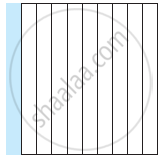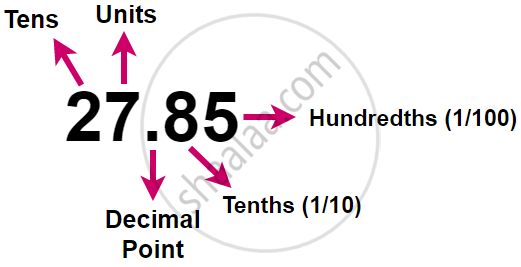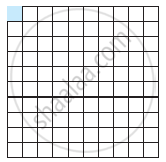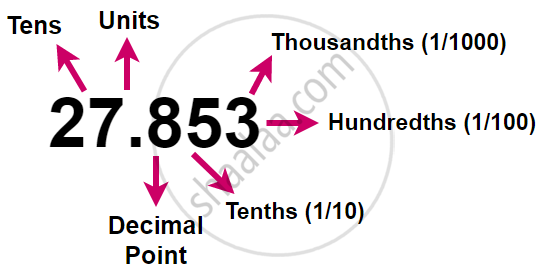# Tenths:

To understand the parts of one whole (i.e., a unit) we represent a unit by a block. One block divide into 10 equal parts means each part is 1/(10) (one-tenth) of a unit. It can be written as 0.1 in decimal notation. The dot represents the decimal point and it comes between the unit's place and the tenths place.

Every fraction with denominator 10 can be written in decimal notation and vice-versa.# Hundredths:

1/100 means 1 part out of 100 parts of a whole.

Hence, the second numbers after the decimal represent the hundredth part of the whole.One block divide into 100 equal parts means each part is 1/(100)(one-hundredth) of a unit. It can be written as 0.01 in decimal notation.# Thousandths:In the place value table, as we go from left to right, the multiplying factor becomes 1/10 of the previous factor.

The place value table can be further extended from hundredths to 1/(10) of hundredths i.e., thousandths (1/(1000)), which is written as 0.001 in decimal notation.

If you would like to contribute notes or other learning material, please submit them using the button below.

### Shaalaa.com

Concept of Tenths, Hundredths and Thousandths in Decimal [00:49:31]
S
0%